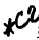Custom Search*C2 C H A P    2,   SEC  V T O R Q U E     P A T H S P A R   4 0 - 4 3 4 0 .    H Y D R A U L I C    S Y S T E M    F U N C T I O N    - DURING   TOW   OR   PUSH   STARTS OF   VEHICLE   (fig.   19) a.   When   it   is   necessary   to   start   the   ve- hicle   engine   by   towing   or   pushing   the   vehicle (refer   to   TM   9-2300-216-10),   the   manual   se- l e c t o r    v a l v e    m u s t    b e    p l a c e d    i n    s e c o n d    g e ar p o s i t i o n. .   T h e    f u n c t i o n    o f    t h e    h y d r a u l i c    s y s t em in   second   gear   during   a   tow-   or   push   -starting o p e r a t i o n    i s    t h e    s a m e    a s    t h a t    d e s c r i b e d    f or second   gear   in   par.   32,   above,   and   illustrated in   fig.   8.   However,   during   such   an   operation (until   the   engine   begins   to   rotate),   the   input d r i v e n    p r e s s u r e    p u m p    c a n    n o t    s u p p l y    m a in p r e s s u r e    t o    t h e    s y s t e m .    T h e    o u t p u t    d r i v en p r e s s u r e    p u m p    m u s t    s u p p l y    m a i n    p r e s s u re until   the   clutches   (output   and   low   range)   engage and   until   torque   is   transmitted   to   the   vehicle engine. c .    T h e    o u t p u t    d r i v e n    p r e s s u r e    p u m p draws   oil,   through   the   sump   screen,   from   the power   train   sump.   The   output   of   the   oil   pump unseats   a   check   valve   and   flows   into   the   cavity s u r r o u n d i n g     t h e     m a i n     o i l     s c r e e n     a s s e m b l y. Thereafter,   the   oil   flow   is   the   same   as   main pressure   oil   flow   for   second   gear   under   any other   condition. .   The   purpose   of   the   check   valve   in   the output   driven   pressure   pump   output   line   is   to prevent   a   back   flow   of   main   pressure   into   the o u t p u t    d r i v e n    p u m p .    I n    n e u t r a l ,    t h e    o u t p ut driven   pump   is   not   rotating   and   there   is   some loss   by   leakage   through   the   pump.   In   reverse gears,   the   pump   rotates   in   a   reverse   direc- tion   and   would   (except   for   the   check   valve) a c t u a l l y    p u m p    o i l    o u t    o f    t h e    m a i n    p r e s s u re line   and   return   it   to   the   sump. Section   V.   TORQUE   PATHS   THROUGH   POWER   TRAIN 4 1 .     G E N E R AL .   Figure   20   is   a   schematic   view   of   the power   train   without   the   output   drive   assem- blies.   All   of   the   principal   components   through which   torque   flows   are   identified.   Figure   21 is   a   cutaway   view   of   the   left-output   drive   as- sembly,   colored   to   indicate   the   torque   path. Subsequent   figures,   22   through   34,   are   colored schematic   views,   illustrating   the   torque   paths for   the   conditions   indicated   in   each   view.   Ar- rows   on   components   indicate   the   direction   of rotation   of   such   components. .   E x p l a n a t i o n s    o f    h y d r a u l i c    a c t i o n s u n d e r    c o r r e s p o n d i n g    c o n d i t i o n s    a r e    i n    p a r s . 28   through   40   of   this   chapter. c .    T o    a v o i d    n e e d l e s s    r e p e t i t i o n ,    r e f e r- e n c e s    a r e    m a d e    t o    p r e c e d i n g    e x p l a n a t i o ns where    applicable. 4 6 4 2 .    T O R Q U E    P A T H    -    I N P U T    T R A N S F ER GEARING   (fig.   20) Torque   is   transmitted   from   the   engine   to the   input   transfer   gearing   by   a   splined   coup- l i n g    s h a f t .    T w o    i d l e r    g e a r s    t r a n s m i t    t o r q ue from   the   input   transfer   drive   gear   to   the   con- v e r t e r    c o v e r    d r i v e    g e a r .    T h e    i n p u t    r o t a t i on (at   coupling   shaft)   is   counterclockwise   when viewed   from   the   left   side   of   the   power   train. Rotation   of   the   torque   converter   is   clockwise, T h e r e    i s    a    s p e e d    i n c r e a s e    r a t i o    o f    0 . 6 1 1    to 1.000   between   the   engine   and   torque   converter. 43. TORQUE   PATHS   -   TORQUE C O N V E R T E R    A N D    L O C K UP CLUTCH   (fig.   20) .   Torque   is   transmitted   from   the   input transfer   gearing   to   the   range   gearing   by   either the   torque   converter   or   lockup   clutch.   When the   lockup   clutch   is   released,   torque   is   trans-Integrated Publishing, Inc. - A (SDVOSB) Service Disabled Veteran Owned Small Business